# Imputed Interest## What is Imputed Interest?

Imputed Interest is basically interest income if one person grants money to other people at zero rates of interest; but the same is considered by IRS to tax the Interest component on the assumption that if the lender would have been a financial institution, it would have charged some interest on the amount lent.

### Types of Imputed Interest

Types of Imputed Interest are linked to the types of loans granted. So, there are different types of loans against which imputed Interest can be computed. These are:

1. Gift Loans: The most famous of its kind when money is granted by father to son or within family members or between friends. However, please note, the money given to the spouse is not considered for imputed Interest.
2. Shareholders Loan: It is the case when a loan is granted by corporate to its shareholders at zero cost to the shareholders
3. Intercorporate Loan: Here, the imputed interest is computed on loan granted by one organization to its sister concern.
4. Loans to Reduce Tax Liability: Here, loans are granted deliberately by one party to another so as to make another person pay interest. It reduces the taxable income of the payer.
5. Zero-Coupon Bonds (ZCB): In this case, bonds are issued at a discount to the face value. No interest is paid during the tenor of the bond. The face value is the amount that the investor receives from the bond issuer at the end of the period. However, the difference between the issue price and the face value of the bond is the net inflow for the investor. Hence, imputed interest is computed on the value of investment i.e. discounted issue price.

However, the IRS exempts a few imputed interests. These are as follows:

• Loans wherein they are subsidized i.e. subsidy granted by government as per need basis.
• No-interest schemes of automobile sectors or no-interest in credit card scheme.
• Relocation loan granted by an employer to his employee
• Gift loans of less than \$ 10,000, unless it is used for buying income-producing assets.
• Loans of less than \$ 10,000 by a corporation to a shareholder are also exempt if the corporate entity can prove that the arrangement was not made for tax avoidance purposes.
• Loans received from a foreign country that would otherwise be not subject to tax laws of the domestic country.

### Components of Imputed Interest

The word “imputed interest” means the implicit or obvious interest one should receive for lending the money. The typical components include:

#### 1. AFR Rate

It means Applicable Federal Rate. It is the base rate used by the Internal Revenue System (IRS), to compare with the Actual Interest charged between the parties. Basically, the IRS published three AFRs viz short-term (three years or less), mid-term (up to nine years) and long-term (more than nine years). The 3-year, 9-year and more-than-9 years are annualized by IRS for ease of computation of imputed Interest. The annualized rate is as follows (January 2019):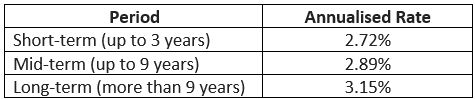Please note that the rates keep on changing as required by the circumstances.

#### 2. Actual Interest Rate

This is the actual rate of interest agreed between the parties. Using this rate, actual Interest is paid by the borrower. However, imputed Interest comes into play when the actual rate is less than AFR.

#### 3. Base Amount

This is the actual amount lent by the lender. Actual Interest is paid on this amount. AFR is also applied to this amount.

#### 4. Taxable Component

This is the difference between the actual Interest amount and the imputed Interest amount. This difference is treated taxable income of the lender party

### Examples of Imputed Interest (With Excel Template)

Let’s take an example to understand the calculation of the Imputed Interest in a better manner.

You can download this Imputed Interest Excel Template here – Imputed Interest Excel Template

Formula

• Step 1: Find the AFR
• Step 2: Compare the AFR with an actual rate
• Step 3: If the actual rate is less than AFR, then the difference is taxable

#### Example #1 – Zero Coupon Bond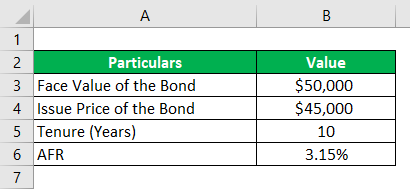Solution:

Step 1: Compute the Differential Income

Differential Income is calculated as

Differential Income = Face Value – Issue Price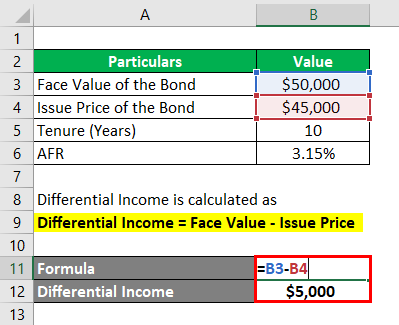• Differential Income = \$50,000 – \$45,000
• Differential Income = \$5,000

Step 2: Compute the actual rate of return (per annum)

Actual Interest Rate is calculated using the formula given below

Actual Interest Rate = (Face Value / Issue Price) 1/n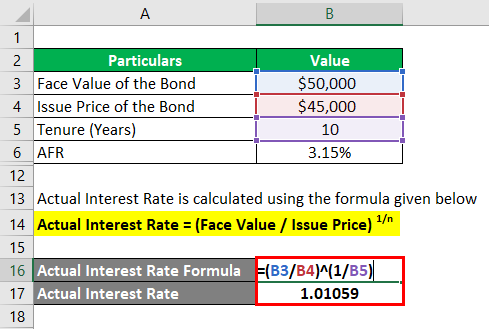• Actual Interest Rate = (\$50,000 / \$45,000) (1/10)
• Actual Interest Rate = 1.01059

Actual Rate of Return is calculated as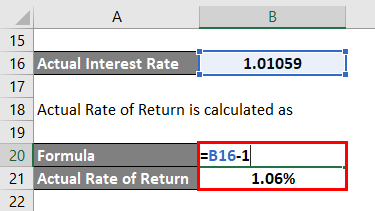• Actual Rate of Return = 1.01059 – 1
• Actual Rate of Return = 1.06%

Step 3: Find the AFR

• For our purpose AFR = 3.15%

Step 4: Imputed Interest

Imputed Interest is calculated as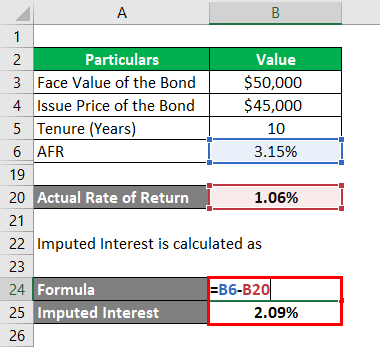• Imputed Interest = 3.15 % – 1.06 %
• Imputed Interest = 2.09%

Imputed Interest Income for Year 1 is calculated as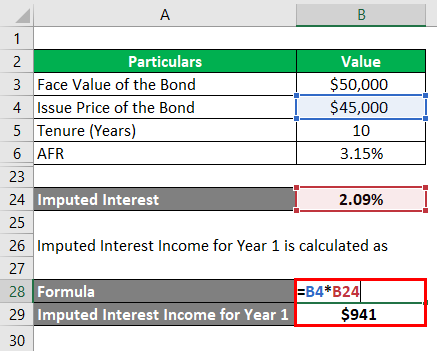•  Imputed Interest Income for Year 1 = \$ 45,000 * 2.09%
• Imputed Interest Income for Year 1 = \$ 941

Actual Interest received for Year 1 = NIL

Step 5: Taxable Income for Year 1

• Taxable Income for Year 1 = \$ 941

Step 6: Closing Balance

Closing Balance is calculated as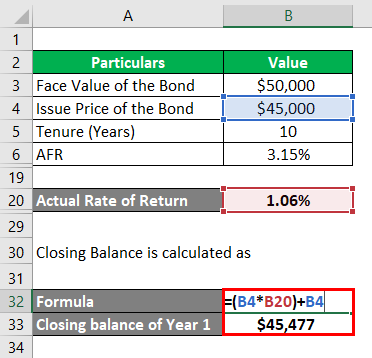• Closing Balance of Year 1 = (45000 * 1.06% ) + 45000 + 477
• Closing Balance of Year 1 = 45477

Step 7: Year 2 onwards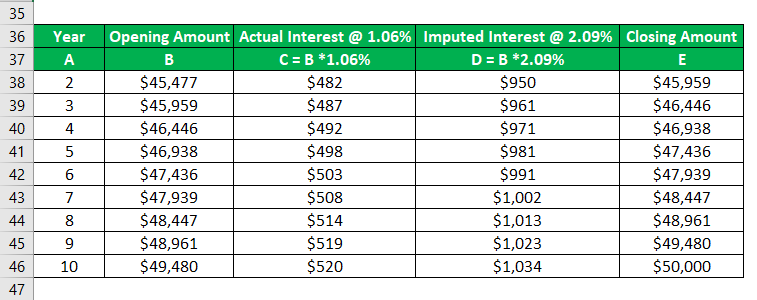#### Example #2 – Inter-Family Loan

Let’s say, a father gives an amount of \$ 60,000 to his son for education purposes for a period of 2 years. Obviously, no interest will be charged. The implied AFR is 2.72%.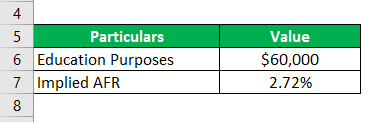Solution:

Taxable Income of Father is calculated as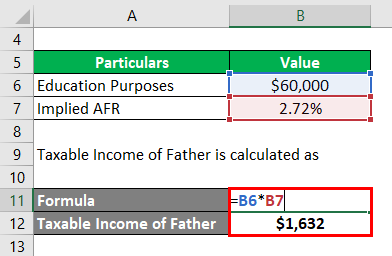• Taxable Income of Father = \$ 60,000 * 2.72%
• Taxable Income of Father = \$1,632

### Advantages and Limitations of Imputed Interest

Advantages and limitations of imputed interest are given below:

• It increases the revenue of IRS
• It helps identify the fraudulent parties.
• It helps identify the transactions that crystalize imputed interest.
• It increases the compliances with IRS
• No hidden surprises for lenders, at the time filing the tax return
• It makes the lenders to tax-plan in advance

#### Limitations

1. If the actual rate is more than AFR, then no immunity is granted to the lender. It means higher income is taxable in case of lower AFR.
2. All transactions may not be identified by the IRS.

Important points to be noted for changes imputed interest:

• The applicable federal rate should be correctly ensured every year
• Computed interest should be offered for tax in the respective years

### Conclusion

On a closing note, Imputed interest is the “should be amount” or “fair value interest”, that is expected to flow between parties involved. Accordingly, this concept also applies to certain bonds that don’t pay interest. For example, zero-coupon bonds (ZCB) are issued at discount than the face value of the bond. Hence, AFR is applied to the actual amount paid for the bond. Further, the difference is added to the taxable income of the lender or bond issuer. Accordingly, tax is payable on the increased amount.

### Recommended Articles

This is a guide to Imputed Interest. Here we discuss the introduction and components of imputed interest along with examples and its types. You may also look at the following articles to learn more –

1. Difference Between Interest vs Dividend
2. Examples of Accrued Interest
3. Formula for Real Interest Rate
4. Difference Between Nominal vs Real Interest Rates

The post Imputed Interest appeared first on EDUCBA.

This post first appeared on Best Online Training & Video Courses | EduCBA, please read the originial post: here